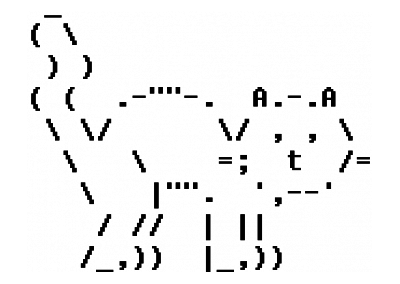# The ASCII tableOriginally I wanted to write about Unicode, but I have realized that it is better to start out with the basics, and you can’t get more basic than the ASCII table.

As computers can only represent two kind of values — true or false — some clever folks back in the middle of the twentieth century constructed a table where they gave meaning to some combination of zeros and ones. That’s how we got this nice table of characters that we actively use even today.

The original ASCII table was 7-bit based. That means that it used only 7 zeros/ones to represent a character. For example to write the character “A” you would send the following down the wire

``````100 0001
``````

Notice the way I formatted the above code — yes, the little space between the first three characters and the last four. There is a reason behind it, namely you can decide the kind of character you are dealing with using only the first three symbols.

# Patterns in the table #

The ASCII table can be divided in 8 logical segments.

Three symbols at the beginning of the code and each of them can have
2 states. That gives us 2 to the power of 3. Hence 8 segments.

The first two segment — that begins with 000 and 001 — contain the control keys that tells the code interpreter specific command such as: start a new line, delete the previous character and similar actions.

The third and fourth segment — that begin with 010 and 011 — contain mathematical and punctuation characters like +, -, !, numbers and the space character.

The fifth, sixth, seventh and eight segment contain mainly the English alphabet, where the 5th and the 6th contains the uppercase, and the 6th and 7th contain the lowercase characters. All the characters are ordered alphabetically.

# Symmetry in the table #

We can get clever and use the fact that the characters are ordered alphabetically. For example if I want to encode the character “D” and I know that uppercase characters start in the 5th section and that we start counting sections from 0

• 4th section in binary is 100
• D is the 4th character, and in binary 0100

The ASCII code for “D” is 100 0100

If now I want to change it to lowercase I can just switch the control codes to the 6th section and get the lowercase “d”

• 6th section, zero based index is 5, in binary 110
• D is the 4th character, and in binary 0100

The ASCII code for “d” is 110 0100

Notice it is only ONE bit different from it’s uppercase code

# Summary #

The ASCII table is an engineering masterpiece that endured the test of time. It’s simplicity and symmetry gives us a great example of organizing complex structures, that proved to be quite useful in a time when we had to enter and recognize these characters manually.